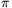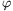Mathematical and Physical Journal
for High Schools
Issued by the MATFUND Foundation
 Already signed up? New to KöMaL?

#Problem A. 380. (October 2005)

A. 380. The convex n-sided polygon K lies in the interior of a unit square. Show that it is possible to select three vertices of the polygon that form a triangle of smaller area thanunits.

(5 pont)

Deadline expired on November 15, 2005.

Solution. We prove that there exist two adjacent sides of K which form a sufficiently small triangle.

K is convex and is a subset of the unit square. Therefore its perimeter p is at most 4.

Denote the length of the ith side of K by di and let the angle between the ith and (i+1)th sides be-i. Sincethere exists an index i for which.

Consider the triangle determnined by the ith and (i+1)th sides; its area is### Statistics:

 15 students sent a solution. 5 points: Dücső Márton, Erdélyi Márton, Gyenizse Gergő, Hujter Bálint, Jankó Zsuzsanna, Kisfaludi-Bak Sándor, Kónya 495 Gábor, Nagy 224 Csaba, Paulin Roland, Tomon István. 4 points: Bogár 560 Péter. 1 point: 3 students. 0 point: 1 student.

Problems in Mathematics of KöMaL, October 2005